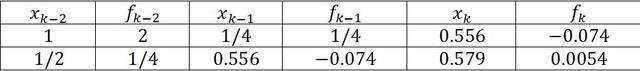# Secant Method Minimum Estimation

• roam

## Homework Statement

I am having some trouble understanding the following solved problem:

Use two iterations of the Secant method to estimate where ##x^2 -x## has a local minimum. Start with ##x^{(0)} =1##, ##x^{(1)} =1/2.#### Homework Equations

Secant iteration:

$$x_k = x_{k-1} - \frac{x_{k-1} - x_{k-2}}{f'_{k-1} - f'_{k-2}}$$

## The Attempt at a Solution

So I understand that for the minimum we need to solve at the derivative of the function, which is

$$3x^2 -1$$

Using the given initial values ##x^{(0)} =1##, ##x^{(1)} =1/2,## the first iteration of the Secant method becomes

$$x^{(3)} = \frac{1}{2} - \frac{(1/2)- 1}{(3(1/2)^2 -1) - (3(1)^2 -1)} = 0.2778$$

So, how did they get ##0.556## for the first iteration?

Also, why did they use ##1/4## instead of ##1/2## as ##x_{k-1}##?

I can't really follow the given answer, so I am wondering if there is an error in the solution. Any help is greatly appreciated.

I have learned a different secant method. Please check it out.

In your calculation, however, in fact you correctly use the function, not the derivative .

 my mistake. Not the function nor the derivative. The derivative of ##x^2-x## is ##2x-1## !

But you do forget to multiply with f(1/2).
And you confuse yourself by calling it x(3)

•roam
Thank you so much for the explanation, it makes perfect sense now. I got the right answer, but I believe there is a typo in the solution in that ##x_{k-1}## in the first iteration should be ##1/2## instead of ##1/4.##

I think you've got it right ! Well observed and no wonder you were a bit disoriented•roam